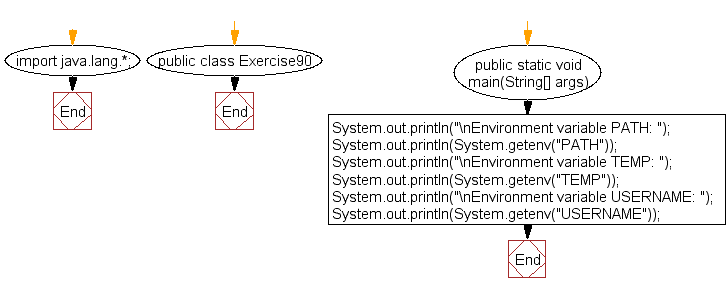﻿ Java: Get the value of the environment variable# Java Exercises: Get the value of the environment variable PATH, TEMP, USERNAME

## Java Basic: Exercise-90 with Solution

Write a Java program to get the value of the environment variable PATH, TEMP, USERNAME.

Sample Solution:

Java Code:

``````import java.lang.*;
public class Exercise90 {
public static void main(String[] args)
{
// gets the value of the specified environment variable "PATH"
System.out.println("\nEnvironment variable PATH: ");
System.out.println(System.getenv("PATH"));

// gets the value of the specified environment variable "TEMP"
System.out.println("\nEnvironment variable TEMP: ");
System.out.println(System.getenv("TEMP"));

// gets the value of the specified environment variable "USERNAME"
}
}
```
```

Sample Output:

```Environment variable PATH:
/usr/local/sbin:/usr/local/bin:/usr/sbin:/usr/bin:/sbin:/bin:/usr/games
:/usr/local/games

Environment variable TEMP:
null

null
```

Flowchart:Java Code Editor:

What is the difficulty level of this exercise?

Test your Programming skills with w3resource's quiz.

﻿

## Java: Tips of the Day

countOccurrences

Counts the occurrences of a value in an array.

Use Arrays.stream().filter().count() to count total number of values that equals the specified value.

```public static long countOccurrences(int[] numbers, int value) {
return Arrays.stream(numbers)
.filter(number -> number == value)
.count();
}
```

Ref: https://bit.ly/3kCAgLb